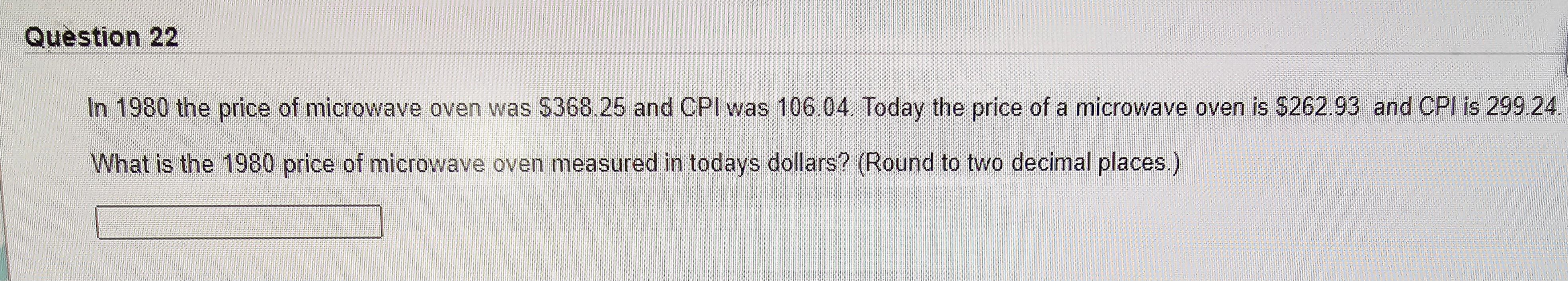Home / Expert Answers / Economics / in-1980-the-price-of-microwave-oven-was-368-25-and-cpi-was-106-04-today-the-price-of-a-microwave-pa950

# (Solved): In 1980 the price of microwave oven was $368.25 and CPI was 106.04. Today the price of a microwave ...In 1980 the price of microwave oven was$368.25 and CPI was 106.04. Today the price of a microwave oven is \$262.93 and CPI is 299.24.

What is the 1980 price of microwave oven measured in todays dollars? (Round to two decimal places.)

In 1980 the price of microwave oven was and CPI was 106.04 . Today the price of a microwave oven is and CPI is 299.24 What is the 1980 price of microwave oven measured in todays dollars? (Round to two decimal places.)

We have an Answer from Expert